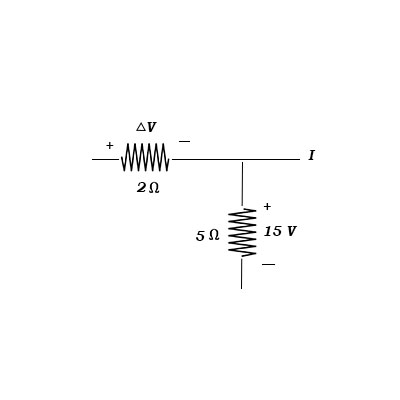# In the figure below, what is the current in the wire to the right of the junction? Suppose that ...

## Question:

In the figure below, what is the current in the wire to the right of the junction? Suppose that {eq}\Delta V = 10 V {/eq}. Express your answer as an integer and include the appropriate units. Enter the positive value if the current is directed to the right and the negative value if current is directed to the left.## Electric Current:

Electric current defines the movement of electric charge carried by electrons in an electrical circuit. The standardized unit in which the current is measured is Ampere. From ohm's law, the current varies linearly with voltage.

Given Data

• {eq}\Delta V = 10\;{\rm{V}} {/eq}.

Current through {eq}2\;{\rm{\Omega }} {/eq} resistor is,

{eq}{I_1} = \dfrac{{\Delta V}}{2} {/eq}

Substituting the values in above expression.

{eq}\begin{align*} {I_1} &= \dfrac{{10}}{2}\\ {I_1} &= 5\;{\rm{A}} \end{align*} {/eq}

Current flowing through {eq}5\;{\rm{\Omega }} {/eq} resistance is,

{eq}\begin{align*} {I_2} &= \dfrac{{15}}{5}\\ {I_2} &= 3\;{\rm{A}} \end{align*} {/eq}

Applying Kirchhoff's current rule at the junction.

{eq}{I_3} = {I_2} - {I_1} {/eq}

Here, {eq}{I_3} {/eq} is the current to the right of junction.

Substituting the values in above expression.

{eq}\begin{align*} {I_3} &= 3 - 7\\ {I_3} &= - 4\;{\rm{A}} \end{align*} {/eq}

As, the value of current is negative. Thus, the current is leaving the junction.